I have restarted my OpenBSD journey about a month ago mostly to try out writing software with `pledge(2)` and `unveil(2)`. It’s actually the second time I have an OpenBSD system, but the last time I was too young to appreciate it. I especially like how straight forward is to get started after having difficulties getting wifi to work and exFAT being not shipped by default and VFAT sticks being a thing of the past… But once I got all the firmware blobs and kernel rebuilt, it was really straight forward.

I decided to try to ressurrect my old netbook, which is this thing (I’d give you the output of `pcidump -v`, but currently that panics the kernel; I suspect it’s because a particular Intel controller does a bunch of stuff, and a couple of them are not configured, and the kernel function to dump all of them involves some address mappings which probably fail for the not configured ones). The funny thing is that they used to ship a 32bit OS on a 64bit system to get it to “run better” and use less memory. Sticking with the theme, yes, I have the i386 build of OpenBSD.

The reason that laptop was retired was mostly because the battery died, and because it was slow and barely a functional computer when it was brand spanking new in 2009. Anyway, I have since acquired quite a collection of laptops and desktops, so I don’t need to depend on it for speed, power or battery life.

I’ve since found a new battery, which, compared to the specs, actually gives me a couple of hours extra battery life. Next would be swapping out the hard drive, since these things fail over time. The CPU doesn’t seem to overheat at all, so I don’t feel like re-thermal pasting it. The fan could probably use some cleaning, but it looks like I have to take the whole danged thing appart to get to it, and that’s a problem for future me.

Today, let’s focus on replacing the hard drive with a brand new modern SSD. I like SSDs in laptop because it reduces the amount of moving parts. It’s a bit of a side-grade (foreshadowing…), since we’ll be switching from 160GB to 120GB.

The list of things we need to do today:

2. Benchmark the old disk
3. Prepare the new disk
4. Copy everything to the new disk and check things are there
5. Test boot with root from the new disk
6. Install the bootloader to the new disk
7. Swap the disks
8. Boot from the new disk for real and benchmark the new disk
9. Be proud of self.

## 1. Acquire a SATA-to-USB adapter.

I got a “Spacer USB 3.0 laptop External HDD Rack”, which included a case to put a disk with its adapter into, and a pretty sweet faux leather case(?) to put it in. I was only interested in the SATA adapter, but this was at the sweet spot between “cheap” and “good reviews” over on the local market.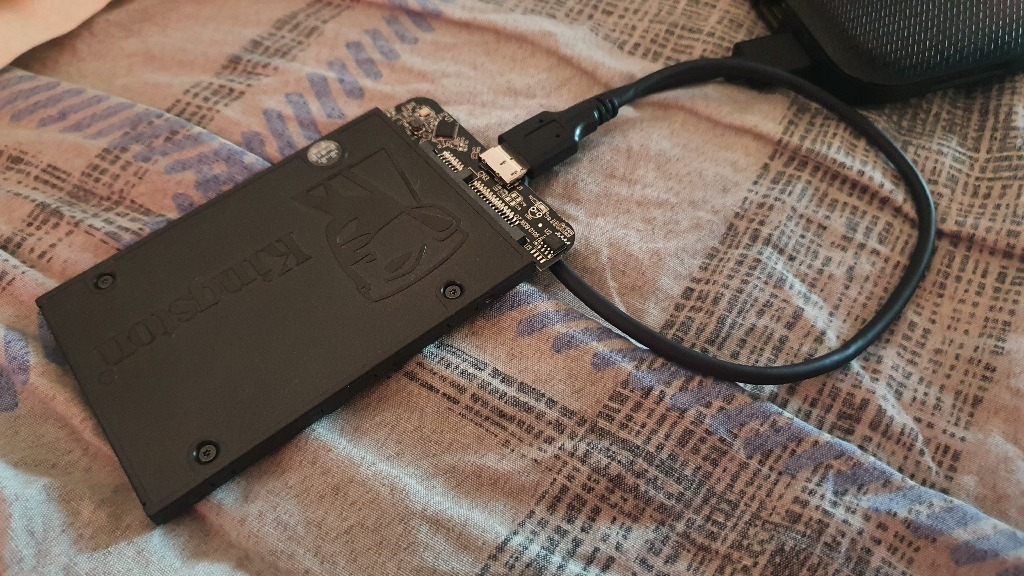Done!

## 2. Benchmark the old disk

It works about as well as a 2.5” disk from ‘09. See benchmarks at the end.

## 3. Prepare the new disk

First things first, what is my new disk? Let’s plug it in, and the console will tell us it’s `sd2`. Cool.

Let’s start with `fdisk(8)`. I expect a single giant “partition” on the old disk.

``````~> doas fdisk sd0
Disk: sd0       geometry: 19457/255/63 [312581808 Sectors]
Offset: 0       Signature: 0xAA55
Starting         Ending         LBA Info:
#: id      C   H   S -      C   H   S [       start:        size ]
-------------------------------------------------------------------------------
0: 00      0   0   0 -      0   0   0 [           0:           0 ] unused
1: 00      0   0   0 -      0   0   0 [           0:           0 ] unused
2: 00      0   0   0 -      0   0   0 [           0:           0 ] unused
*3: A6      0   1   2 -  19457  80  63 [          64:   312581744 ] OpenBSD
``````

Indeed. Let’s replicate that on the new disk with the magic “initialize disk”.

``````doas fdisk -iy sd2
``````
``````~> doas fdisk sd2
Disk: sd2       geometry: 14593/255/63 [234441647 Sectors]
Offset: 0       Signature: 0xAA55
Starting         Ending         LBA Info:
#: id      C   H   S -      C   H   S [       start:        size ]
-------------------------------------------------------------------------------
0: 00      0   0   0 -      0   0   0 [           0:           0 ] unused
1: 00      0   0   0 -      0   0   0 [           0:           0 ] unused
2: 00      0   0   0 -      0   0   0 [           0:           0 ] unused
*3: A6      0   1   2 -  14593  80  62 [          64:   234441583 ] OpenBSD
``````

Nice. Cylinders, heads and sectors are starting to rear their ugly heads, but this is something we tollerate when it comes to hard drives on PCs.

Let’s check the existing disk label:

``````disklabel sd0
# /dev/rsd0c:
type: SCSI
disk: SCSI disk
label: Hitachi HTS54501
duid: db0061b2fe09e6a0
flags:
bytes/sector: 512
sectors/track: 63
tracks/cylinder: 255
sectors/cylinder: 16065
cylinders: 19457
total sectors: 312581808
boundstart: 64
boundend: 312581808
drivedata: 0
16 partitions:
#                size           offset  fstype [fsize bsize   cpg]
a:          2097152               64  4.2BSD   2048 16384 12960 # /
b:          2599656          2097216    swap                    # none
c:        312581808                0  unused
d:          8388576          4696896  4.2BSD   2048 16384 12960 # /tmp
e:         12539328         13085472  4.2BSD   2048 16384 12960 # /var
f:         12582912         25624800  4.2BSD   2048 16384 12960 # /usr
g:          2097152         38207712  4.2BSD   2048 16384 12960 # /usr/X11R6
h:         41943040         40304864  4.2BSD   2048 16384 12960 # /usr/local
i:          6291456         82247904  4.2BSD   2048 16384 12960 # /usr/src
j:         12582912         88539360  4.2BSD   2048 16384 12960 # /usr/obj
k:        211459520        101122272  4.2BSD   2048 16384 12960 # /home
``````

And `df`:

``````> df -h
Filesystem     Size    Used   Avail Capacity  Mounted on
/dev/sd0a      986M    515M    422M    55%    /
/dev/sd0k     97.7G   13.6G   79.2G    15%    /home
/dev/sd0d      3.9G    220K    3.7G     0%    /tmp
/dev/sd0f      5.8G    2.2G    3.4G    39%    /usr
/dev/sd0g      986M    248M    688M    26%    /usr/X11R6
/dev/sd0h     19.4G    3.8G   14.6G    21%    /usr/local
/dev/sd0j      5.8G    241M    5.3G     4%    /usr/obj
/dev/sd0i      2.9G    1.8G   1014M    64%    /usr/src
/dev/sd0e      5.8G   32.3M    5.5G     1%    /var
``````

Originally, it was a bog standard automagic partitioning that OpenBSD provides. No complaints so far.

``````disklabel sd2
16 partitions:
#                size           offset  fstype [fsize bsize   cpg]
c:        234441647                0  unused

``````

The numbers `disklabel(8)` shows are sector counts, and a sector is 512 bytes (because diskabel said so, though in my experience, drive firmware almost always reports 512; maybe it was part of the original IBM PC spec? Who knows; it turns out the original spec lasted a good 3 years before hard drives became bigger than the max representable size, he he).

To convert, you can use `dc(1)` or `bc(1)` or `expr(1)` or a calculator or your brain. I use `dc`:

``````pseudo> dc
2k «GB» 2 1024 1024 * * * p       => blocks
2k «BLOCKS» 2 / 1024 / 1024 / p   => GB
``````

We can even alias this in tcsh:

``````> alias GBtoS 'echo "5k \!:1 2 1024 1024 * * * p" | dc'
> alias StoGB 'echo "5k \!:1 2 / 1024 / 1024 / p" | dc'
> GBtoS 1
2097152
> StoGB 2097152
1.00000
``````

I wouldn’t stick that in my user’s rc, but it’s handy while we’re figuring out these counts. The trick is to divide/multiply by 2 and ignore the last 6 digits.

Back to disk labeling, let’s try automatic mode:

``````disklabel -E sd2
sd2> A
sd2*> p
OpenBSD area: 64-234441647; size: 234441583; free: 31
#                size           offset  fstype [fsize bsize   cpg]
a:          2097152               64  4.2BSD   2048 16384     1 # /
b:          2599568          2097216    swap
c:        234441647                0  unused
d:          8388576          4696800  4.2BSD   2048 16384     1 # /tmp
e:         12539168         13085376  4.2BSD   2048 16384     1 # /var
f:         12582912         25624544  4.2BSD   2048 16384     1 # /usr
g:          2097152         38207456  4.2BSD   2048 16384     1 # /usr/X11R6
h:         33889696         40304608  4.2BSD   2048 16384     1 # /usr/local
i:          6291456         74194304  4.2BSD   2048 16384     1 # /usr/src
j:         12582912         80485760  4.2BSD   2048 16384     1 # /usr/obj
k:        141372960         93068672  4.2BSD   2048 16384     1 # /home
``````

Close, but let’s say I do not like that. I think I’ll round down `/var` to 4GB and `/usr/local` to 16GB. That should give me almost 2 gigs extra for `/home`. I could also decide I want to consolidate `a`, `f` and `g` and sum up their sizes into a single big a partition, but nah.

Delete everything with `z` and add each individual partition with `a x`:

``````> disklabel -E sd2
Label editor (enter '?' for help at any prompt)
sd2> z
sd2*> w
sd2> p
OpenBSD area: 64-234441647; size: 234441583; free: 234441583
#                size           offset  fstype [fsize bsize   cpg]
c:        234441647                0  unused
sd2> a a
offset: 
size:  2097152
FS type: [4.2BSD]
sd2*> a b
offset: 
size:  2599568
FS type: [swap] swap
sd2*> a d
offset: 
size:  8388576
FS type: [4.2BSD]
sd2*> a e
offset: 
size:  8388608
FS type: [4.2BSD]
sd2*> a f
offset: 
size:  12582912
FS type: [4.2BSD]
sd2*> a g
offset: 
size:  2097152
FS type: [4.2BSD]
sd2*> a h
offset: 
size:  33554432
FS type: [4.2BSD]
sd2*> a i
offset: 
size:  6291456
FS type: [4.2BSD]
sd2*> a j
offset: 
size:  12582912
FS type: [4.2BSD]
sd2*> a k
offset: 
size: 
FS type: [4.2BSD]
sd2*> p
OpenBSD area: 64-234441647; size: 234441583; free: 31
#                size           offset  fstype [fsize bsize   cpg]
a:          2097152               64  4.2BSD   2048 16384     1
b:          2599568          2097216    swap
c:        234441647                0  unused
d:          8388544          4696800  4.2BSD   2048 16384     1
e:          8388608         13085344  4.2BSD   2048 16384     1
f:         12582912         21473952  4.2BSD   2048 16384     1
g:          2097152         34056864  4.2BSD   2048 16384     1
h:         33554432         36154016  4.2BSD   2048 16384     1
i:          6291456         69708448  4.2BSD   2048 16384     1
j:         12582912         75999904  4.2BSD   2048 16384     1
k:        145858816         88582816  4.2BSD   2048 16384     1
sd2*> w
``````
``````doas disklabel sd2
# /dev/rsd2c:
type: SCSI
disk: SCSI disk
label: sage 3639S
duid: f511cf504ce61e02
flags:
bytes/sector: 512
sectors/track: 63
tracks/cylinder: 255
sectors/cylinder: 16065
cylinders: 14593
total sectors: 234441647
boundstart: 64
boundend: 234441647
drivedata: 0

16 partitions:
#                size           offset  fstype [fsize bsize   cpg]
a:          2097152               64  4.2BSD   2048 16384     1
b:          2599568          2097216    swap
c:        234441647                0  unused
d:          8388544          4696800  4.2BSD   2048 16384     1
e:          8388608         13085344  4.2BSD   2048 16384     1
f:         12582912         21473952  4.2BSD   2048 16384     1
g:          2097152         34056864  4.2BSD   2048 16384     1
h:         33554432         36154016  4.2BSD   2048 16384     1
i:          6291456         69708448  4.2BSD   2048 16384     1
j:         12582912         75999904  4.2BSD   2048 16384     1
k:        145858816         88582816  4.2BSD   2048 16384     1
``````

Now, create all partitions…

``````foreach d ( sd2{a,d,e,f,g,h,i,j,k} )
newfs \$d
end
``````

Let’s test a partition, because why not:

``````jakkal# mount /dev/sd2a /mnt
jakkal# cd /mnt
/mnt# ls
/mnt# ls -a
.  ..
/mnt# df -h
Filesystem     Size    Used   Avail Capacity  Mounted on
/dev/sd0a      986M    515M    422M    55%    /
/dev/sd0k     97.7G   13.6G   79.2G    15%    /home
/dev/sd0d      3.9G    220K    3.7G     0%    /tmp
/dev/sd0f      5.8G    2.2G    3.4G    39%    /usr
/dev/sd0g      986M    248M    688M    26%    /usr/X11R6
/dev/sd0h     19.4G    3.8G   14.6G    21%    /usr/local
/dev/sd0j      5.8G    241M    5.3G     4%    /usr/obj
/dev/sd0i      2.9G    1.8G   1014M    64%    /usr/src
/dev/sd0e      5.8G   32.3M    5.5G     1%    /var
/dev/sd2a      986M    2.0K    937M     0%    /mnt
/mnt# echo hi > hi
/mnt# cat hi
hi
``````

Cool.

``````cd / ; umount /mnt
``````

Let’s try to copy stuff.

``````/# mount /dev/sd2e /mnt
/# df -h
Filesystem     Size    Used   Avail Capacity  Mounted on
/dev/sd0a      986M    515M    422M    55%    /
/dev/sd0k     97.7G   13.6G   79.2G    15%    /home
/dev/sd0d      3.9G    198K    3.7G     0%    /tmp
/dev/sd0f      5.8G    2.2G    3.4G    39%    /usr
/dev/sd0g      986M    248M    688M    26%    /usr/X11R6
/dev/sd0h     19.4G    3.8G   14.6G    21%    /usr/local
/dev/sd0j      5.8G    248M    5.3G     4%    /usr/obj
/dev/sd0i      2.9G    1.8G   1014M    64%    /usr/src
/dev/sd0e      5.8G   32.2M    5.5G     1%    /var
/dev/sd2e      3.9G    2.0K    3.7G     0%    /mnt

/# cd /mnt
/mnt# dump -0f - /var/ | restore -rf -
DUMP: Dumping sub files/directories from /var
DUMP: Dumping file/directory /var/
DUMP: Date of this level 0 dump: Tue Oct 18 20:09:06 2022
DUMP: Date of last level 0 dump: the epoch
DUMP: Dumping /dev/rsd0e (/var) to standard output
DUMP: mapping (Pass I) [regular files]
DUMP: mapping (Pass II) [directories]
DUMP: estimated 42447 tape blocks.
DUMP: Volume 1 started at: Tue Oct 18 20:09:07 2022
DUMP: dumping (Pass III) [directories]
DUMP: dumping (Pass IV) [regular files]
DUMP: 35015 tape blocks
DUMP: Date of this level 0 dump: Tue Oct 18 20:09:06 2022
DUMP: Volume 1 completed at: Tue Oct 18 20:09:18 2022
DUMP: Volume 1 took 0:00:11
DUMP: Volume 1 transfer rate: 3183 KB/s
DUMP: Date this dump completed:  Tue Oct 18 20:09:18 2022
DUMP: Average transfer rate: 3183 KB/s
DUMP: DUMP IS DONE
``````

The OpenBSD internet sources recommend `dump(8)` and `tar(1)`. `dump` works on the raw device (e.g. `/dev/rsd0a`) which will allow us to skip mounting some things once we get to copying everything. `cp` won’t work because you can’t tell it to stay on just one filesystem nor to exclude files, which complicates things.

`dump` creates a restoresymtable which is used for incremental restores; -0 effectively means full back each higher level means “backup everything since the last backup of a lower level”, so you would restore backup level 0 and continue with 1, 2… up to how many levels your backup policy has. But that’s besides the point, I’m only using it to copy paste the file systems one time.

``````/mnt# ls -lh restoresymtable
-rw-------  1 root  wheel   766K Oct 18 20:09 restoresymtable
``````

Anyway, let’s re-wipe this; we need to go to singleuser mode to do this properly. Singleuser mode mounts `/` readonly and nothing else, which is a great time to duplciate everything without missing any work.

``````# cd / ; umount /mnt
# newfs sd2e
/dev/rsd2e: 4096.0MB in 8388608 sectors of 512 bytes
21 cylinder groups of 202.50MB, 12960 blocks, 25920 inodes each
super-block backups (for fsck -b #) at:
160, 414880, 829600, 1244320, 1659040, 2073760, 2488480, 2903200, 3317920,
3732640, 4147360, 4562080, 4976800, 5391520, 5806240, 6220960, 6635680,
7050400, 7465120, 7879840, 8294560,
``````

## 4. Copy everything

Reboot into single user mode with nothing mounted and nothing going on and a readonly `/`, so that we can get a good snapshop.

`reboot`, then `boot -s` at the `boot>` prompt.

It prompts for a shell; I’ve had `tcsh` compiled statically and installed in `/bin`, so I have that available to me. So I type in `/bin/tcsh`.

Before we go any further, we need a read-write `/tmp`. Slice `sd0d` will not get cloned, since that is `/tmp`, and I don’t have that much RAM on my system either (1GB), so I might as well mount the real `sd0d`:

``````mount /tmp
``````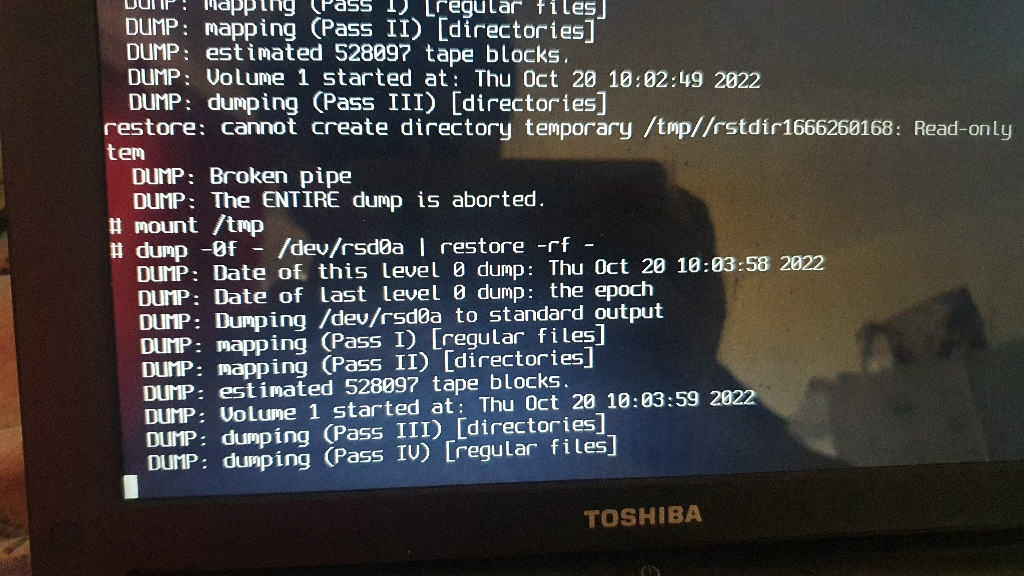If you had a single `/`, you would have to use `mount_mfs(8)` to get a ramdisk on `/tmp` (if you hadn’t done that already, in which case `mount /tmp`).

Start with `/` to get it out of the way, since it has an annoying extra step:

``````mount /dev/sd2a /mnt
cd /mnt
dump -0f - /dev/rsd0a | restore -rf -
``````

Note, in single user mode, it doesn’t exactly actually really know what device `/` is, so we have to tell it. It’s nice `dump` takes raw devices, since it saves us from having to mount all the partitions on the original disk, which means less opportunities to have our disks diverge.

That’s the copy, but `/etc/fstab` contains the DUID of the old disk. If you scroll back up, both were mentioned, so we can do a `s/re/place/`. If you’re following closely, we don’t have `/usr` nor `/usr/local` mounted, which means most fun things aren’t available, though, thankfully, good ol’ reliable `ed(1)` is. If you know a bit of `sed(1)` and a bit of `vi(1)`, you know `ed`.

``````> ed /mnt/etc/fstab
%s/db0061b2fe09e6a0/f511cf504ce61e02/
%n
wq
``````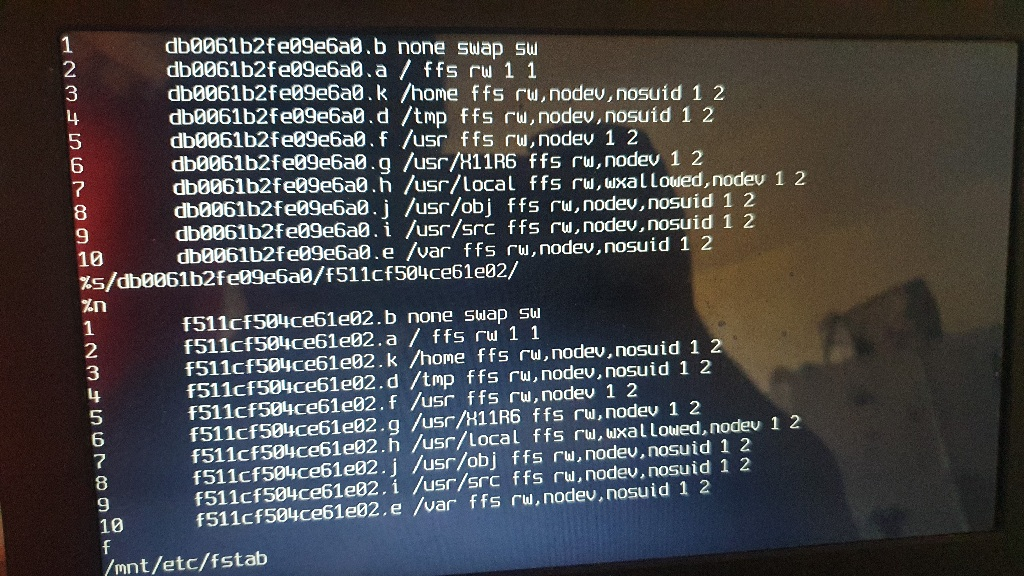`%` means “address all lines” (like `vi`), `s///` means replace (like `sed` and `vi`), `n` means “print with line numbers” (like `ex(1)`; there’s also the basic `p` for print, but I like my line numbers), and then there’s `wq` (like `vi`) to save and quit.

Fun fact, in ed’s implementation, `q` is actually a modifier of `w` in this, and you can’t generally chain commands.

Anyway, enough about `ed` (my favourite text editor; btw, I recommend the book Ed Mastery). We’re done with `/`.

``````cd /
umount /mnt
``````

Dump creates `restoresymtable` which we don’t need since we only ever restore the backup, but it helps to make sure when we boot into this later, it actually mounts the correct disk’s partitions (e.g. we didn’t forget to adjust the fstab). We’ll delete those later.

For the remaining partitions… Skip `b` (swap), skip `c` (full disk), skip `d` (`/tmp`), continue from `e` through the end.

repeat:

``````mount /dev/sd2X /mnt
cd /mnt
dump -0f - /dev/rsd0X | restore -rf -
cd /
umount
``````

It’s important to be in `/mnt` before running `restore`, `restore` will dump stuff in its cwd. If you’ve consolidated some partitions, make sure you are in the correct `/mnt` subdirectory before running `restore`.

Oh, and if you’ve split partitions, then make sure all the target slices are moutned before running `restore`, so that data ends up in the correct place.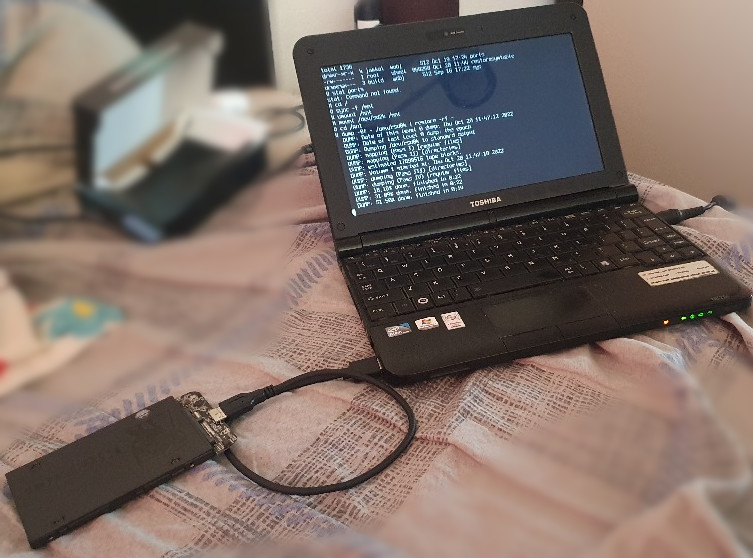## 5. Test new disk has all the data for it to boot to the same desktop you know and love

Let’s test the boot. `reboot`, then `boot sd2a:/bsd`

And I get dropped to a TTY. Weird. I log in, see `xenodm` failed inexplicably, try to start, X comes up for a split second, then crashes, and the logs aren’t helpful. So I did some googling related to an unrelated error message, and some fellow recommended that some packages need to be installed, so I try to run `pkg_add(1)` and there it is, `/tmp` has the wrong permissions. No idea how, no idea why, but this is something I need to remember to check in the future on any system that has gremlins. It was `0755`, it should be `1777`, so, as root, `chmod 1777 /tmp`. Now X starts. But I’d rather just reboot and make sure it works… Again, `boot sd2a:/bsd`.

Aaaaand it works fine. X start up. `vim` starts up. Yay!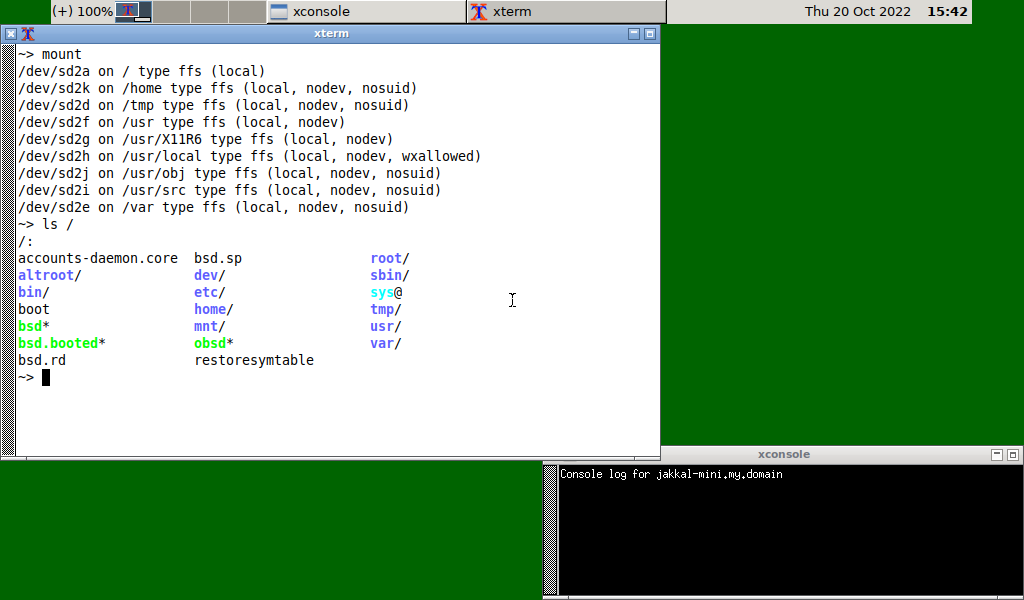(hmmm… when did that core dump get there?)

We’re not quite done with the disk yet, it has no boot loader. We need to use `installboot(8)`. The `i386` manpage says to just run it, so…

``````installboot sd2
``````

If you want to know more about the 3 (or more if not on PC?) stages of booting, refer to the man pages.

Another round of boot tests; this time, we need to kindly ask our BIOS to boot off of USB. Spoilers: it works fine.

## 7. Swap the disks

Cool. Let’s swap the disks IRL…

Looking at the bottom of the laptop, it looks like there are a couple of Torx screws to get out of the way (because I have to justify having bought a couple of sets of Torx heads over the years), and the maintenance manual confirms this. The maintenance manual also says to “carefully remove the Hard Drive cover as shown in the figure” but it doesn’t actually show how. If you have such a laptop and you don’t want to break the cover (which includes shielding), you’re supposed to remove if inward-to-outward (pull up from the VGA port side) while gently pulling it towards the longer edge (that frees up the last tab on the shorter edge), and then you can pull it out towards the VGA port side.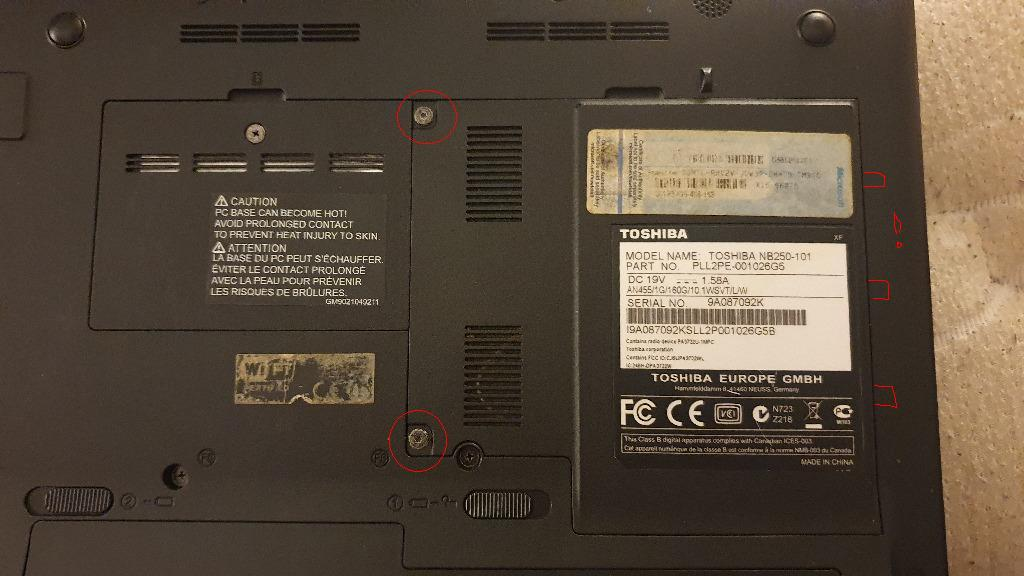The old disk just slides out (fun fact – the maintenance manual mentioned every other paragraph to not press down on the “middle of the hard drive” to not damage the platter or head). It’s in some sort of caddy, imprisoned by 4 philips head screws. Those come right out, SSD goes in, SSD slides in, cover is reinstalled, we should be good to go.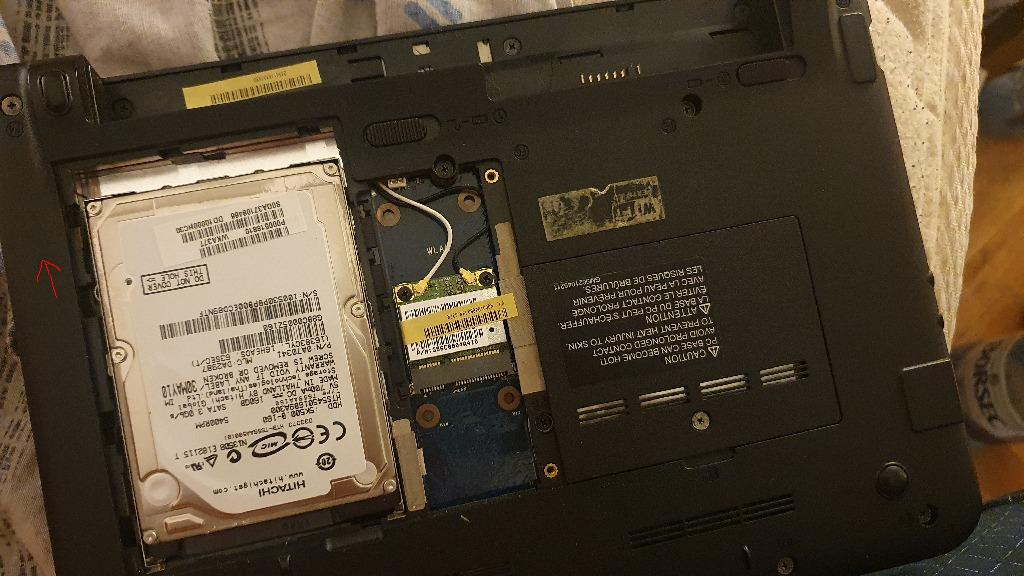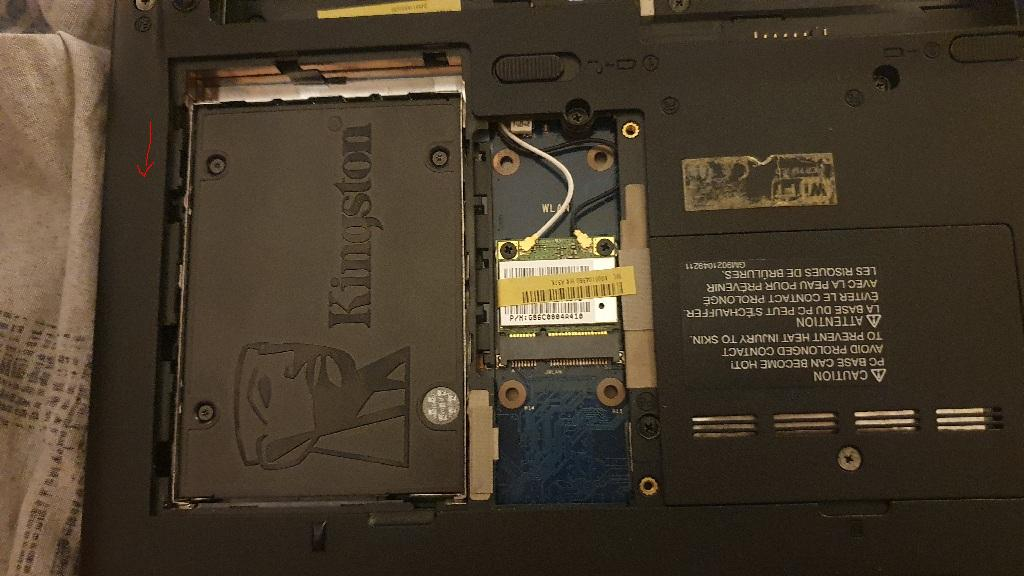## 8. Boot from the new disk for real and benchmark the new disk

Aaaaaand we run into a bit of a wall. My Bios doesn’t “see” the SSD. Much googling has given me some pointers, like flip between “IDE” and “AHCI” SATA modes in the BIOS, unfortunately, I cannot seem to enter BIOS settings (it just reboots when the BIOS setup menu is supposed to show up; and I could only find someone on the internet with the same problem and no solution). This laptop used to have a Windows 7 Starter installation with a Windows Vista utility called Toshiba HWSetup, which is long gone. I’ll try to get windows on some USB drive to try to get some version of that installed and maybe it lets me switch between those modes, or at least disable PXEBOOT or maybe something else (foreshadowing…). Anyway, I’ve tried Win PE and Hiren’s BootCD and obvious stuff, that utility won’t work there, and I’ve tried 5 versions, and I don’t have a spare disk for a real install right now (I’m still hanging on to the old Hitachi in case this SSD thing doesn’t work out… foreshadowing).

So, I know the drive boots off of the SATA-to-USB interface, I know it boots off of the SATA-to-USB interface without the old hard drive in there, and I know I can boot off of a usb thumb drive (which is how I installed OpenBSD on this thing in the first place).

Booting off of a USB pen drive with the installation media on it (`install71.img`), it does not give me the option to boot off of the SSD either (it only lists hd0 which is the USB stick itself). But, if I continue to boot it and press U for `(U)pdate`, it does prompt me about `sd0`. Escaping to a shell and fiddling about, I can tell the USB stick itself is now `sd2`. So everything seems fine once the kernel is booted.

Looking through `boot(8)`, I see we can ask the bootloader to ask where the root partition is. Let’s try that! Reboot, at the prompt type `boot -a`. When prompted, tell it `sd0a` for root and `sd0b` for swap. It starts to boot, but hangs after starting `ntpd`. Okay, maybe `/boot.rd` isn’t the best image to actually load the operating system (which is the default image for the installation media).

Let’s try with `/bsd`. Reboot, say `boot /bsd -a`, when prompted for the root partition, tell it `sd0a` for root and `sd0b` for swap and… it almost boots correctly. There are no additional vttys (or whatever BSD calls them) installed, so X won’t start. Network seems to work. There were a whole lot more devices showing up as “not configured” in `dmesg`, but maybe that’s because the kernel on the installation media doesn’t have a whole much of anything in it. Maybe the installer configures the kernel it’s installing resulting in a kernel that can actually configure all of the devices.

Since we’re now sort-of booted into my usual system, let’s hack!

``````mount /dev/sd2a /mnt
mv /mnt/bsd /mnt/bsd.installer
cp /bsd /mnt/bsd
``````

Reading about `boot.conf` in the man pages, I see I can specify some things to have a few characters less to type:

``````mv /mnt/etc/boot.conf /mnt/etc/boot.conf.old
cat <<EOF > /mnt/etc/boot.conf
set image /bsd
set howto -a
EOF
``````

Now let’s get out of here:

``````cd /
sync -f /mnt
umount /mnt
reboot
``````

I’ve searched the whole wide world (wide web) if I can tell the bootloader to have `root on sd0a swap on sd0b dump on sd0b`, but it turns out you can only do that when recompiling the kernel. I’ll wait on that since recompiling the kernel on this ancient netbook takes at least two hours. But, now that it’s back at the `boot>` prompt, let’s hit enter and see what happens.

It reads the new `/etc/boot.conf` and uses the new `/bsd` kernel which we copied over from our SSD (and ultimately from the original disk), and then asks for the root device: `sd0a`, enter, aaaaand… Yes, we’re booted off of the SSD, X is running, I can log in, everything is mounted, and we can remove the USB stick.

I am pleasantly surprised OpenBSD can do this (though I know of an HP-UX “mini” in a particular basement that can only boot off of a very specific floppy, so it’s not unheard of in the world of UNXIen). I need to try this on Linux at some point, it probably requires grub and the kernel on the USB fub, which then magically becomes the `/boot` partition or something like that; or maybe grub can already read the SSD at that point?

So, we are in 2022 and I have a system that effectively needs a boot “floppy”. Now, I just need to gather the necessary willpower to recompile the kernel (again) with `conf bsd root on sd0a swap on sd0b dump on sd0b` and I don’t have to type `sd0a<CR><CR>` every time I boot now.

The only additional annoyance is that if I hibernate the system, the BIOS knows it was hibernated and disables the boot menu and for some strange reason `lan` (PXE) takes precedence over USB. This means I need to `CTRL+ALT+DEL` out of the failing PXE boot 3 times until it will let me tell it to use the USB. Then, it proceeds to boot asking for root, and then proceeds to detect the hibernation image and load it. And everything is fine afterwards.

Why would I torture myself this way with a boot floppy-actually-usb-drive? I have a feeling that old hard drive will give up the ghost one of these days, while the SSD definitely has a few years’ worth of life in it. And I’ll have to check the benchmarks, maybe we do get some speed improvements (more foreshadowing!).

Finally, delete those `restoresymtable` files: (I’m feeling courages and using shell expansions as root, woooo!)

``````doas rm -f {/,/var/,/home/,/usr/,/usr/X11R6/,/usr/local/,/usr/obj/,/usr/src/}restoresymtable
``````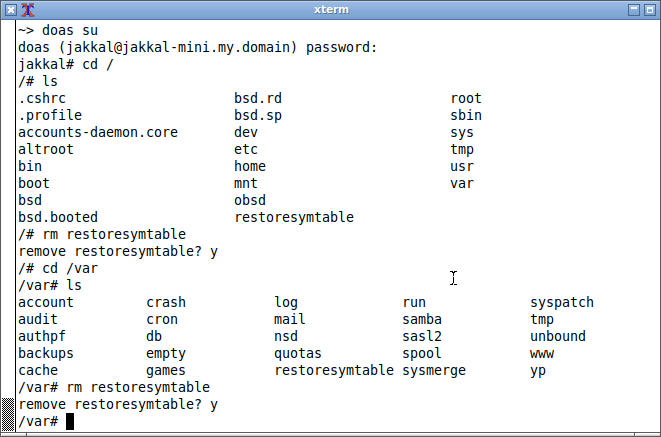## 9. Benchmarks?

Now that I can proudly boot my system again, albeit with a few extra steps, a magic incantation and by sacrificing a goat, let’s see what I’ve gained, if anything.

I ran this:

``````doas fio --name=read --iodepth=1 --rw=read --bs=4k --direct=0 --size=512M --numjobs=2 --runtime=240 --group_reporting
doas fio --name=write --iodepth=1 --rw=write --bs=4k --direct=0 --size=512M --numjobs=2 --runtime=240 --group_reporting
doas fio --name=randrw --iodepth=1 --rw=randrw --bs=4k --direct=0 --size=128MB --numjobs=2 --runtime=240 --group_reporting
``````

I’m not good at disk benchmarks, but usually they test sequential and random reads and writes. 4k should be a block (I don’t know how to check), `numjobs` is 2 because I have a single hyperthreaded core, `iodepth=1` because I’m not sure if higher depths are supported without `libaio` or if it matters on SATA. Shrugs, at least I ran the same tests on both disks.

Oh, if you run those, it will create files named something like `randrw.0.0` which are 128/512MB. Don’t forget to delete them.

Here’s a table:

spinny disk 10.3 MB/s 30.3 MB/s (?) 483 KB/s 257-479 KB/s read, 280-482 KB/s write
ssd 73.0 MB/s 55.5 MB/s 45 MB/s 13.4 MB/s read, 13.5 MB/s write

Note: Sequential write on the spinny disk is a bit weird. In general, writes seem to have gone quicker. I didn’t have any notable processes running, and repeating the test gave consistently better write results. Maybe the disk was just getting old?

To me, the only relevant benchmark is `random reads and writes`, since that is the closest to telling me where the bottleneck would be when compiling & building big- or many projects. I’m most happy about the greatly reduced latency (which I care about mcuh more than GBps sequential transfer speeds). And with 13ish MB/s in random RW, and 1033 MHz RAM, I’m pretty sure it’s the CPU, which is practically glued to the motherboard.

Overall, moving to SSD was a net upgrade. Well, if you disregard the fact that I need a USB boot key and system upgrades have a couple of more steps to it. The main reason I wanted to replace the old disk was to know that I can (the old disk was at least 13 years old, which was waaaay past its intended life time) and because I wanted to preemptively do so before it died. The speed boost is a bonus, the convoluted boot process is a malus. But hey, at least now doing full disk encryption wouldn’t be an annoyance since I need the boot stick anyway, amirite? :smile:

I should probably go about recompiling that kernel to have `root on ... swap on ... dump on ...`, it might genuinely take a lot less time this time around, but I’ll wait until I upgrade to 7.2, otherwise it’s one pointless extra kernel recompile.

Next, the raw output of `fio` if it means anything to you.

### Before

Here’s the before (with the old spinny disk installed):

``````read: (g=0): rw=read, bs=(R) 4096B-4096B, (W) 4096B-4096B, (T) 4096B-4096B, ioengine=psync, iodepth=1
...
fio-3.26
Jobs: 2 (f=2): [R(2)][100.0%][r=25.2MiB/s][r=6446 IOPS][eta 00m:00s]
read: (groupid=0, jobs=2): err= 0: pid=2138416428: Wed Oct 19 21:19:38 2022
clat (usec): min=23, max=88329, avg=718.63, stdev=4160.79
lat (usec): min=29, max=88335, avg=726.04, stdev=4160.66
clat percentiles (usec):
|  1.00th=[   25],  5.00th=[   26], 10.00th=[   27], 20.00th=[   27],
| 30.00th=[   32], 40.00th=[   33], 50.00th=[   34], 60.00th=[   35],
| 70.00th=[   39], 80.00th=[   41], 90.00th=[   85], 95.00th=[  388],
| 99.00th=, 99.50th=, 99.90th=, 99.95th=,
| 99.99th=
bw (  KiB/s): min= 3238, max=109169, per=99.44%, avg=10526.60, stdev=5844.51, samples=394
iops        : min=  808, max=27291, avg=2630.99, stdev=1461.18, samples=394
lat (usec)   : 50=88.09%, 100=2.75%, 250=0.40%, 500=4.18%, 750=0.08%
lat (usec)   : 1000=1.21%
lat (msec)   : 2=0.25%, 4=0.15%, 10=0.17%, 20=0.95%, 50=1.76%
lat (msec)   : 100=0.01%
cpu          : usr=3.26%, sys=8.55%, ctx=15871, majf=0, minf=6
IO depths    : 1=100.0%, 2=0.0%, 4=0.0%, 8=0.0%, 16=0.0%, 32=0.0%, >=64=0.0%
submit    : 0=0.0%, 4=100.0%, 8=0.0%, 16=0.0%, 32=0.0%, 64=0.0%, >=64=0.0%
complete  : 0=0.0%, 4=100.0%, 8=0.0%, 16=0.0%, 32=0.0%, 64=0.0%, >=64=0.0%
issued rwts: total=262144,0,0,0 short=0,0,0,0 dropped=0,0,0,0
latency   : target=0, window=0, percentile=100.00%, depth=1

Run status group 0 (all jobs):
READ: bw=10.3MiB/s (10.8MB/s), 10.3MiB/s-10.3MiB/s (10.8MB/s-10.8MB/s), io=1024MiB (1074MB), run=99064-99064msec
``````

#### Sequential write:

``````write: (g=0): rw=write, bs=(R) 4096B-4096B, (W) 4096B-4096B, (T) 4096B-4096B, ioengine=psync, iodepth=1
...
fio-3.26
write: Laying out IO file (1 file / 512MiB)
write: Laying out IO file (1 file / 512MiB)
Jobs: 2 (f=2): [W(2)][97.1%][w=26.3MiB/s][w=6735 IOPS][eta 00m:01s]
write: (groupid=0, jobs=2): err= 0: pid=1676831276: Wed Oct 19 21:28:13 2022
write: IOPS=7754, BW=30.3MiB/s (31.8MB/s)(1024MiB/33807msec); 0 zone resets
clat (usec): min=28, max=152399, avg=213.93, stdev=2318.41
lat (usec): min=35, max=152407, avg=223.02, stdev=2318.37
clat percentiles (usec):
|  1.00th=[   36],  5.00th=[   43], 10.00th=[   47], 20.00th=[   48],
| 30.00th=[   51], 40.00th=[   57], 50.00th=[   73], 60.00th=[   97],
| 70.00th=[  119], 80.00th=[  128], 90.00th=[  159], 95.00th=[  180],
| 99.00th=[  277], 99.50th=[  537], 99.90th=, 99.95th=,
| 99.99th=
bw (  KiB/s): min=19416, max=44486, per=100.00%, avg=31041.66, stdev=2323.90, samples=134
iops        : min= 4853, max=11119, avg=7759.90, stdev=580.96, samples=134
lat (usec)   : 50=29.27%, 100=33.37%, 250=36.07%, 500=0.79%, 750=0.03%
lat (usec)   : 1000=0.01%
lat (msec)   : 2=0.01%, 4=0.01%, 10=0.05%, 20=0.16%, 50=0.22%
lat (msec)   : 100=0.03%, 250=0.01%
cpu          : usr=11.37%, sys=37.57%, ctx=2689, majf=0, minf=1
IO depths    : 1=100.0%, 2=0.0%, 4=0.0%, 8=0.0%, 16=0.0%, 32=0.0%, >=64=0.0%
submit    : 0=0.0%, 4=100.0%, 8=0.0%, 16=0.0%, 32=0.0%, 64=0.0%, >=64=0.0%
complete  : 0=0.0%, 4=100.0%, 8=0.0%, 16=0.0%, 32=0.0%, 64=0.0%, >=64=0.0%
issued rwts: total=0,262144,0,0 short=0,0,0,0 dropped=0,0,0,0
latency   : target=0, window=0, percentile=100.00%, depth=1

Run status group 0 (all jobs):
WRITE: bw=30.3MiB/s (31.8MB/s), 30.3MiB/s-30.3MiB/s (31.8MB/s-31.8MB/s), io=1024MiB (1074MB), run=33807-33807msec
``````

``````randread: (g=0): rw=randread, bs=(R) 4096B-4096B, (W) 4096B-4096B, (T) 4096B-4096B, ioengine=psync, iodepth=1
...
fio-3.26
Jobs: 2 (f=2): [r(2)][100.0%][r=552KiB/s][r=138 IOPS][eta 00m:00s]
randread: (groupid=0, jobs=2): err= 0: pid=1071443244: Wed Oct 19 21:32:56 2022
clat (usec): min=23, max=88032, avg=18230.89, stdev=14964.63
lat (usec): min=29, max=88039, avg=18237.47, stdev=14964.61
clat percentiles (usec):
|  1.00th=[   27],  5.00th=[   30], 10.00th=[   35], 20.00th=[   37],
| 30.00th=[   39], 40.00th=, 50.00th=, 60.00th=,
| 70.00th=, 80.00th=, 90.00th=, 95.00th=,
| 99.00th=, 99.50th=, 99.90th=, 99.95th=,
| 99.99th=
bw (  KiB/s): min=  214, max=  856, per=99.80%, avg=437.82, stdev=56.06, samples=958
iops        : min=   52, max=  214, avg=109.43, stdev=14.03, samples=958
lat (usec)   : 50=36.07%, 100=0.57%, 250=0.16%, 500=0.02%
lat (msec)   : 4=0.02%, 10=0.38%, 20=5.84%, 50=56.91%, 100=0.03%
cpu          : usr=0.18%, sys=0.49%, ctx=16656, majf=0, minf=5
IO depths    : 1=100.0%, 2=0.0%, 4=0.0%, 8=0.0%, 16=0.0%, 32=0.0%, >=64=0.0%
submit    : 0=0.0%, 4=100.0%, 8=0.0%, 16=0.0%, 32=0.0%, 64=0.0%, >=64=0.0%
complete  : 0=0.0%, 4=100.0%, 8=0.0%, 16=0.0%, 32=0.0%, 64=0.0%, >=64=0.0%
issued rwts: total=26276,0,0,0 short=0,0,0,0 dropped=0,0,0,0
latency   : target=0, window=0, percentile=100.00%, depth=1

Run status group 0 (all jobs):
READ: bw=438KiB/s (448kB/s), 438KiB/s-438KiB/s (448kB/s-448kB/s), io=103MiB (108MB), run=240024-240024msec
``````

``````randrw: (g=0): rw=randrw, bs=(R) 4096B-4096B, (W) 4096B-4096B, (T) 4096B-4096B, ioengine=psync, iodepth=1
...
fio-3.26
randrw: Laying out IO file (1 file / 128MiB)
randrw: Laying out IO file (1 file / 128MiB)
Jobs: 2 (f=2): [m(2)][100.0%][r=648KiB/s,w=712KiB/s][r=162,w=178 IOPS][eta 00m:00s]
randrw: (groupid=0, jobs=2): err= 0: pid=1888610348: Wed Oct 19 21:41:06 2022
clat (usec): min=23, max=2606.6k, avg=15257.28, stdev=48556.36
lat (usec): min=29, max=2606.6k, avg=15263.83, stdev=48556.46
clat percentiles (usec):
|  1.00th=[     27],  5.00th=[     29], 10.00th=[     35],
| 20.00th=[     36], 30.00th=[     37], 40.00th=[     38],
| 50.00th=[     41], 60.00th=[  15401], 70.00th=[  20841],
| 80.00th=[  24511], 90.00th=[  28443], 95.00th=[  32113],
| 99.00th=[ 263193], 99.50th=[ 375391], 99.90th=[ 505414],
| 99.95th=[ 557843], 99.99th=
bw (  KiB/s): min=   16, max=  769, per=100.00%, avg=273.99, stdev=87.06, samples=888
iops        : min=    4, max=  192, avg=68.48, stdev=21.76, samples=888
write: IOPS=64, BW=260KiB/s (266kB/s)(60.9MiB/240020msec); 0 zone resets
clat (usec): min=31, max=4929.2k, avg=15623.81, stdev=64345.23
lat (usec): min=38, max=4929.2k, avg=15630.99, stdev=64345.38
clat percentiles (usec):
|  1.00th=[     39],  5.00th=[     44], 10.00th=[     47],
| 20.00th=[     50], 30.00th=[     53], 40.00th=[     64],
| 50.00th=[     73], 60.00th=[  15664], 70.00th=[  21103],
| 80.00th=[  24511], 90.00th=[  28705], 95.00th=[  32113],
| 99.00th=[ 242222], 99.50th=[ 375391], 99.90th=[ 541066],
| 99.95th=[ 633340], 99.99th=
bw (  KiB/s): min=   16, max=  769, per=100.00%, avg=280.23, stdev=85.96, samples=880
iops        : min=    4, max=  192, avg=70.04, stdev=21.48, samples=880
lat (usec)   : 50=37.28%, 100=18.65%, 250=0.45%, 500=0.02%, 750=0.02%
lat (usec)   : 1000=0.01%
lat (msec)   : 4=0.01%, 10=0.52%, 20=10.71%, 50=29.81%, 100=1.18%
lat (msec)   : 250=0.36%, 500=0.86%, 750=0.12%, 1000=0.01%, 2000=0.01%
lat (msec)   : >=2000=0.01%
cpu          : usr=0.19%, sys=0.58%, ctx=13596, majf=0, minf=3
IO depths    : 1=100.0%, 2=0.0%, 4=0.0%, 8=0.0%, 16=0.0%, 32=0.0%, >=64=0.0%
submit    : 0=0.0%, 4=100.0%, 8=0.0%, 16=0.0%, 32=0.0%, 64=0.0%, >=64=0.0%
complete  : 0=0.0%, 4=100.0%, 8=0.0%, 16=0.0%, 32=0.0%, 64=0.0%, >=64=0.0%
issued rwts: total=15414,15595,0,0 short=0,0,0,0 dropped=0,0,0,0
latency   : target=0, window=0, percentile=100.00%, depth=1

Run status group 0 (all jobs):
READ: bw=257KiB/s (263kB/s), 257KiB/s-257KiB/s (263kB/s-263kB/s), io=60.2MiB (63.1MB), run=240020-240020msec
WRITE: bw=260KiB/s (266kB/s), 260KiB/s-260KiB/s (266kB/s-266kB/s), io=60.9MiB (63.9MB), run=240020-240020msec
``````

I figured I may as well try `iodepth=64` and it didn’t seem to stick. But I did get better results the second time.

``````doas fio --name=randrw --iodepth=64 --rw=randrw --bs=4k --direct=0 --size=128M --numjobs=2 --runtime=240 --group_reporting
randrw: (g=0): rw=randrw, bs=(R) 4096B-4096B, (W) 4096B-4096B, (T) 4096B-4096B, ioengine=psync, iodepth=64
...
fio-3.26
Jobs: 1 (f=1): [m(1),_(1)][72.2%][r=728KiB/s,w=800KiB/s][r=182,w=200 IOPS][eta 01m:33s]
randrw: (groupid=0, jobs=2): err= 0: pid=1735393836: Wed Oct 19 22:00:06 2022
clat (usec): min=22, max=7388.8k, avg=5958.35, stdev=54733.25
lat (usec): min=28, max=7388.8k, avg=5964.81, stdev=54733.33
clat percentiles (usec):
|  1.00th=[    25],  5.00th=[    26], 10.00th=[    28], 20.00th=[    34],
| 30.00th=[    35], 40.00th=[    35], 50.00th=[    36], 60.00th=[    37],
| 70.00th=[    38], 80.00th=[    44], 90.00th=[ 13960], 95.00th=[ 23725],
| 99.00th=[ 89654], 99.50th=, 99.90th=, 99.95th=,
| 99.99th=
bw (  KiB/s): min=   16, max= 4889, per=100.00%, avg=859.73, stdev=406.90, samples=620
iops        : min=    4, max= 1221, avg=214.77, stdev=101.65, samples=620
write: IOPS=117, BW=471KiB/s (482kB/s)(110MiB/240074msec); 0 zone resets
clat (usec): min=27, max=8351.6k, avg=6755.65, stdev=77544.85
lat (usec): min=34, max=8351.6k, avg=6762.72, stdev=77544.93
clat percentiles (usec):
|  1.00th=[     35],  5.00th=[     39], 10.00th=[     42],
| 20.00th=[     45], 30.00th=[     47], 40.00th=[     48],
| 50.00th=[     50], 60.00th=[     57], 70.00th=[     65],
| 80.00th=[     74], 90.00th=[  14353], 95.00th=[  23987],
| 99.00th=[  93848], 99.50th=[ 308282], 99.90th=[ 492831],
| 99.95th=[ 599786], 99.99th=
bw (  KiB/s): min=   16, max= 4969, per=100.00%, avg=860.65, stdev=400.65, samples=633
iops        : min=    4, max= 1241, avg=214.99, stdev=100.09, samples=633
lat (usec)   : 50=66.68%, 100=16.92%, 250=0.41%, 500=0.14%, 750=0.06%
lat (usec)   : 1000=0.01%
lat (msec)   : 2=0.01%, 4=0.12%, 10=2.72%, 20=6.20%, 50=5.20%
lat (msec)   : 100=0.64%, 250=0.30%, 500=0.51%, 750=0.07%, 1000=0.01%
lat (msec)   : 2000=0.01%, >=2000=0.01%
cpu          : usr=0.39%, sys=1.05%, ctx=9246, majf=0, minf=7
IO depths    : 1=100.0%, 2=0.0%, 4=0.0%, 8=0.0%, 16=0.0%, 32=0.0%, >=64=0.0%
submit    : 0=0.0%, 4=100.0%, 8=0.0%, 16=0.0%, 32=0.0%, 64=0.0%, >=64=0.0%
complete  : 0=0.0%, 4=100.0%, 8=0.0%, 16=0.0%, 32=0.0%, 64=0.0%, >=64=0.0%
issued rwts: total=28091,28255,0,0 short=0,0,0,0 dropped=0,0,0,0
latency   : target=0, window=0, percentile=100.00%, depth=64

Run status group 0 (all jobs):
READ: bw=468KiB/s (479kB/s), 468KiB/s-468KiB/s (479kB/s-479kB/s), io=110MiB (115MB), run=240074-240074msec
WRITE: bw=471KiB/s (482kB/s), 471KiB/s-471KiB/s (482kB/s-482kB/s), io=110MiB (116MB), run=240074-240074msec
``````

### After

Here’s the after (with the SSD installed):

``````read: (g=0): rw=read, bs=(R) 4096B-4096B, (W) 4096B-4096B, (T) 4096B-4096B, ioengine=psync, iodepth=1
...
fio-3.26
read: Laying out IO file (1 file / 512MiB)
read: Laying out IO file (1 file / 512MiB)
Jobs: 2 (f=2): [R(2)][93.3%][r=72.2MiB/s][r=18.5k IOPS][eta 00m:01s]
read: (groupid=0, jobs=2): err= 0: pid=1106000428: Fri Oct 21 20:59:33 2022
clat (usec): min=21, max=11117, avg=68.93, stdev=103.54
lat (usec): min=27, max=11125, avg=76.69, stdev=104.33
clat percentiles (usec):
|  1.00th=[   27],  5.00th=[   31], 10.00th=[   32], 20.00th=[   33],
| 30.00th=[   33], 40.00th=[   34], 50.00th=[   36], 60.00th=[   38],
| 70.00th=[   40], 80.00th=[   45], 90.00th=[  219], 95.00th=[  326],
| 99.00th=[  392], 99.50th=[  416], 99.90th=[  474], 99.95th=[  586],
| 99.99th=[ 2212]
bw (  KiB/s): min=70350, max=76199, per=100.00%, avg=74897.37, stdev=639.32, samples=54
iops        : min=17587, max=19049, avg=18723.52, stdev=159.83, samples=54
lat (usec)   : 50=84.54%, 100=3.03%, 250=3.62%, 500=8.73%, 750=0.04%
lat (usec)   : 1000=0.01%
lat (msec)   : 2=0.01%, 4=0.01%, 10=0.01%, 20=0.01%
cpu          : usr=22.91%, sys=62.56%, ctx=23754, majf=8, minf=1
IO depths    : 1=100.0%, 2=0.0%, 4=0.0%, 8=0.0%, 16=0.0%, 32=0.0%, >=64=0.0%
submit    : 0=0.0%, 4=100.0%, 8=0.0%, 16=0.0%, 32=0.0%, 64=0.0%, >=64=0.0%
complete  : 0=0.0%, 4=100.0%, 8=0.0%, 16=0.0%, 32=0.0%, 64=0.0%, >=64=0.0%
issued rwts: total=262144,0,0,0 short=0,0,0,0 dropped=0,0,0,0
latency   : target=0, window=0, percentile=100.00%, depth=1

Run status group 0 (all jobs):
READ: bw=73.0MiB/s (76.6MB/s), 73.0MiB/s-73.0MiB/s (76.6MB/s-76.6MB/s), io=1024MiB (1074MB), run=14021-14021msec
``````

#### Sequential write

``````write: (g=0): rw=write, bs=(R) 4096B-4096B, (W) 4096B-4096B, (T) 4096B-4096B, ioengine=psync, iodepth=1
...
fio-3.26
write: Laying out IO file (1 file / 512MiB)
write: Laying out IO file (1 file / 512MiB)
Jobs: 2 (f=2): [W(2)][100.0%][w=54.6MiB/s][w=14.0k IOPS][eta 00m:00s]
write: (groupid=0, jobs=2): err= 0: pid=1294197548: Fri Oct 21 21:01:30 2022
write: IOPS=14.2k, BW=55.5MiB/s (58.2MB/s)(1024MiB/18445msec); 0 zone resets
clat (usec): min=30, max=32031, avg=90.73, stdev=115.00
lat (usec): min=37, max=32039, avg=106.75, stdev=117.14
clat percentiles (usec):
|  1.00th=[   41],  5.00th=[   46], 10.00th=[   47], 20.00th=[   48],
| 30.00th=[   60], 40.00th=[   72], 50.00th=[   77], 60.00th=[   82],
| 70.00th=[  114], 80.00th=[  135], 90.00th=[  143], 95.00th=[  163],
| 99.00th=[  210], 99.50th=[  219], 99.90th=[  351], 99.95th=[  449],
| 99.99th=[ 2024]
bw (  KiB/s): min=54183, max=61626, per=100.00%, avg=56890.61, stdev=658.86, samples=72
iops        : min=13545, max=15406, avg=14222.39, stdev=164.67, samples=72
lat (usec)   : 50=26.15%, 100=37.23%, 250=36.33%, 500=0.24%, 750=0.02%
lat (usec)   : 1000=0.01%
lat (msec)   : 2=0.01%, 4=0.01%, 10=0.01%, 50=0.01%
cpu          : usr=20.15%, sys=69.26%, ctx=1512, majf=0, minf=1
IO depths    : 1=100.0%, 2=0.0%, 4=0.0%, 8=0.0%, 16=0.0%, 32=0.0%, >=64=0.0%
submit    : 0=0.0%, 4=100.0%, 8=0.0%, 16=0.0%, 32=0.0%, 64=0.0%, >=64=0.0%
complete  : 0=0.0%, 4=100.0%, 8=0.0%, 16=0.0%, 32=0.0%, 64=0.0%, >=64=0.0%
issued rwts: total=0,262144,0,0 short=0,0,0,0 dropped=0,0,0,0
latency   : target=0, window=0, percentile=100.00%, depth=1

Run status group 0 (all jobs):
WRITE: bw=55.5MiB/s (58.2MB/s), 55.5MiB/s-55.5MiB/s (58.2MB/s-58.2MB/s), io=1024MiB (1074MB), run=18445-18445msec
``````

``````randread: (g=0): rw=randread, bs=(R) 4096B-4096B, (W) 4096B-4096B, (T) 4096B-4096B, ioengine=psync, iodepth=1
...
fio-3.26
randread: Laying out IO file (1 file / 128MiB)
Jobs: 1 (f=1): [r(1),_(1)][85.7%][r=32.1MiB/s][r=8212 IOPS][eta 00m:01s]
randread: (groupid=0, jobs=2): err= 0: pid=1068637740: Fri Oct 21 21:02:49 2022
clat (usec): min=21, max=14297, avg=94.65, stdev=125.13
lat (usec): min=27, max=14307, avg=101.70, stdev=125.32
clat percentiles (usec):
|  1.00th=[   25],  5.00th=[   26], 10.00th=[   28], 20.00th=[   34],
| 30.00th=[   34], 40.00th=[   35], 50.00th=[   38], 60.00th=[   41],
| 70.00th=[   51], 80.00th=[  122], 90.00th=[  302], 95.00th=[  326],
| 99.00th=[  388], 99.50th=[  412], 99.90th=[  469], 99.95th=[  498],
| 99.99th=[  922]
bw (  KiB/s): min=45163, max=89291, per=100.00%, avg=63953.70, stdev=7830.61, samples=16
iops        : min=11290, max=22322, avg=15987.70, stdev=1957.58, samples=16
lat (usec)   : 50=69.85%, 100=9.02%, 250=1.34%, 500=19.75%, 750=0.03%
lat (usec)   : 1000=0.01%
lat (msec)   : 2=0.01%, 20=0.01%
cpu          : usr=18.56%, sys=50.41%, ctx=13163, majf=0, minf=2
IO depths    : 1=100.0%, 2=0.0%, 4=0.0%, 8=0.0%, 16=0.0%, 32=0.0%, >=64=0.0%
submit    : 0=0.0%, 4=100.0%, 8=0.0%, 16=0.0%, 32=0.0%, 64=0.0%, >=64=0.0%
complete  : 0=0.0%, 4=100.0%, 8=0.0%, 16=0.0%, 32=0.0%, 64=0.0%, >=64=0.0%
issued rwts: total=65536,0,0,0 short=0,0,0,0 dropped=0,0,0,0
latency   : target=0, window=0, percentile=100.00%, depth=1

Run status group 0 (all jobs):
READ: bw=45.8MiB/s (48.0MB/s), 45.8MiB/s-45.8MiB/s (48.0MB/s-48.0MB/s), io=256MiB (268MB), run=5593-5593msec
``````

``````randrw: (g=0): rw=randrw, bs=(R) 4096B-4096B, (W) 4096B-4096B, (T) 4096B-4096B, ioengine=psync, iodepth=1
...
fio-3.26
randrw: Laying out IO file (1 file / 128MiB)
randrw: Laying out IO file (1 file / 128MiB)
Jobs: 2 (f=2): [m(2)][21.4%][r=10.8MiB/s,w=11.0MiB/s][r=2771,w=2826 IOPS][eta 00Jobs: 2 (f=2): [m(2)][30.8%][r=12.2MiB/s,w=12.4MiB/s][r=3128,w=3179 IOPS][eta 00Jobs: 2 (f=2): [m(2)][38.5%][r=13.6MiB/s,w=13.2MiB/s][r=3493,w=3385 IOPS][eta 00Jobs: 2 (f=2): [m(2)][50.0%][r=13.4MiB/s,w=13.4MiB/s][r=3429,w=3418 IOPS][eta 00Jobs: 2 (f=2): [m(2)][58.3%][r=14.5MiB/s,w=14.7MiB/s][r=3712,w=3752 IOPS][eta 00Jobs: 2 (f=2): [m(2)][66.7%][r=16.1MiB/s,w=16.3MiB/s][r=4122,w=4184 IOPS][eta 00Jobs: 2 (f=2): [m(2)][81.8%][r=15.1MiB/s,w=14.8MiB/s][r=3873,w=3797 IOPS][eta 00Jobs: 1 (f=1): [m(1),_(1)][90.9%][r=11.8MiB/s,w=11.5MiB/s][r=3009,w=2951 IOPS][eta 00m:01s]
randrw: (groupid=0, jobs=2): err= 0: pid=1813041708: Fri Oct 21 21:03:40 2022
clat (usec): min=22, max=50779, avg=218.19, stdev=753.90
lat (usec): min=28, max=50787, avg=225.66, stdev=754.69
clat percentiles (usec):
|  1.00th=[   26],  5.00th=[   28], 10.00th=[   34], 20.00th=[   36],
| 30.00th=[   38], 40.00th=[   42], 50.00th=[   62], 60.00th=[  120],
| 70.00th=[  330], 80.00th=[  355], 90.00th=[  404], 95.00th=[  453],
| 99.00th=[  824], 99.50th=[ 4883], 99.90th=[ 6063], 99.95th=[ 6521],
| 99.99th=
bw (  KiB/s): min=10997, max=20919, per=100.00%, avg=14616.86, stdev=1501.32, samples=33
iops        : min= 2749, max= 5229, avg=3653.57, stdev=375.28, samples=33
write: IOPS=3453, BW=13.5MiB/s (14.1MB/s)(128MiB/9512msec); 0 zone resets
clat (usec): min=26, max=50212, avg=237.08, stdev=762.37
lat (usec): min=34, max=50218, avg=245.18, stdev=762.59
clat percentiles (usec):
|  1.00th=[   37],  5.00th=[   43], 10.00th=[   48], 20.00th=[   51],
| 30.00th=[   61], 40.00th=[   71], 50.00th=[   87], 60.00th=[  141],
| 70.00th=[  343], 80.00th=[  367], 90.00th=[  420], 95.00th=[  474],
| 99.00th=[  832], 99.50th=[ 4883], 99.90th=[ 5997], 99.95th=[ 7504],
| 99.99th=
bw (  KiB/s): min=10824, max=21233, per=100.00%, avg=14714.54, stdev=1499.95, samples=33
iops        : min= 2705, max= 5308, avg=3677.98, stdev=375.05, samples=33
lat (usec)   : 50=31.70%, 100=24.08%, 250=6.15%, 500=34.35%, 750=2.21%
lat (usec)   : 1000=0.76%
lat (msec)   : 2=0.06%, 4=0.09%, 10=0.57%, 20=0.01%, 50=0.02%
lat (msec)   : 100=0.01%
cpu          : usr=11.15%, sys=35.93%, ctx=25241, majf=0, minf=2
IO depths    : 1=100.0%, 2=0.0%, 4=0.0%, 8=0.0%, 16=0.0%, 32=0.0%, >=64=0.0%
submit    : 0=0.0%, 4=100.0%, 8=0.0%, 16=0.0%, 32=0.0%, 64=0.0%, >=64=0.0%
complete  : 0=0.0%, 4=100.0%, 8=0.0%, 16=0.0%, 32=0.0%, 64=0.0%, >=64=0.0%
issued rwts: total=32682,32854,0,0 short=0,0,0,0 dropped=0,0,0,0
latency   : target=0, window=0, percentile=100.00%, depth=1

Run status group 0 (all jobs):
READ: bw=13.4MiB/s (14.1MB/s), 13.4MiB/s-13.4MiB/s (14.1MB/s-14.1MB/s), io=128MiB (134MB), run=9512-9512msec
WRITE: bw=13.5MiB/s (14.1MB/s), 13.5MiB/s-13.5MiB/s (14.1MB/s-14.1MB/s), io=128MiB (135MB), run=9512-9512msec
``````